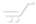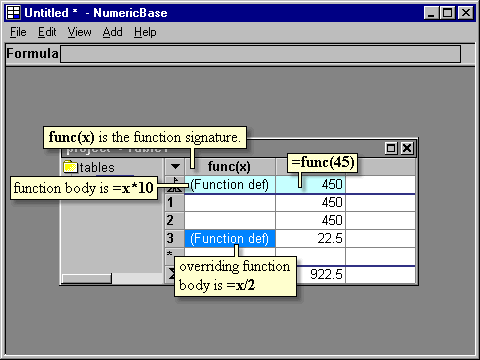# User functions

User functions
NumericBase let you define your own functions. To do this, you need to add a new column and give it a name that can behave as a "function signature".

Function signature
A function signature is composed of the function name followed by zero or more parameter names separated by commas and surrounded by parentheses. For example: user_function(x,y,z). The parameter names can not repeat themselves. For example : user_function(x,x) is not a valid function signature.

The body
The body of the user function is defined as a formula in the base row. You can override this body at any other row.

For example, the following screenshot shows a user function named func(x). The body of the function, shown in the base row e of this column, is =x*10. On the other column there is a formula that uses this function =func(45). The function body is overridden in row number 2 to be =x/2. The corresponding row that uses this function is now 22.5 instead of 450.Screenshot: using a user function